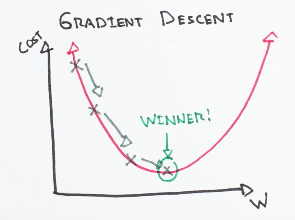# Gradient descent: The core of neural networks

Friday, March 2, 2018

As discussed in the post linear algebra and deep learning, the optimization is the third and last step in solving image classification problem in deep learning. It helps us find the values of weights W and bias b that minimizes the loss function.### The strategy

We use the concept of following the gradient to find the optimal pairs of W and b i.e. we can compute the best direction along which we should change our weight vectors that is mathematically guaranteed to be the direction of the steepest descent. This direction is found out by the gradient of the loss function.

### Computation

There are two ways to compute the gradient:

• Numerical gradient: The numerical gradient is very simple to compute using the finite difference approximation, but the downside is that it is approximate and that it is very computationally expensive to compute. We use the following expression to calculate numerical gradient:
$\displaystyle{\frac{df(x)}{dx} = \lim \limits_{h \to 0} \frac{f(x + h) - f(x)}{h}}$
• Analytical gradient: The second way to compute the gradient is analytically using Calculus, which allows us to derive a direct formula for the gradient (no approximations) that is also very fast to compute.

Unlike the numerical gradient it can be more error prone to implement, which is why in practice it is very common to compute the analytic gradient and compare it to the numerical gradient to check the correctness of your implementation. This is called a gradient check.

Now that we can compute the gradient of the loss function, the procedure of repeatedly evaluating the gradient and then performing a parameter update is called gradient descent.

This simple loop is at the core of all Neural Network libraries.

When the training data is large, it’s computationally expensive and wasteful to compute the full loss function over the entire training set to perform a single parameter update. Thus, a simple solution of using the batches of training data is implemented. This batch is then used to perform parameter update.

The extreme case of this is a setting where the mini-batch contains only a single example. This process is called Stochastic Gradient Descent (SGD) (or on-line gradient descent).

### Conclusion

Gradient descent is one of many optimization methods, namely first order optimizer, meaning, that it is based on analysis of the gradient of the objective. In order to calculate gradients efficiently, we use backpropagation. Consequently, in terms of neural networks it is often applied together with backprop to make an efficient updates.